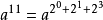#### 快速幂是什么？11的二进制是1011
11 = 2³×1 + 2²×0 + 2¹×1 + 2º×1

#### 如何编写快速幂代码？

``` //快速幂1（数字较小）：
#include<bits/stdc++.h>
using namespace std;
long long f(long long n,long long m)
{
//求的是m个n相乘，这里n是一个正整数
if(m==)return ;
else if(m==)return n;
else if(m%==)return f(n*n,m/);//偶数时的降幂
return f(n*n,m/)*n;//奇数时的降幂
}
int main()
{
long long n,m;
cin>>n>>m;
cout<<f(n,m);
return ;
}```
``` //快速幂2（数字较大）：
#include<bits/stdc++.h>
using namespace std;
int k;
long long f(long long n,long long m)
{
//求的是m个n相乘，这里n是一个正整数
if(m==)return ;
else if(m==)return n%k;
else if(m%==)return f((n%k)*(n%k),m/)%k;//偶数时的降幂
return f((n%k)*(n%k),m/)*(n%k)%k;//奇数时的降幂
}
int main()
{
long long n,m;
cin>>n>>m>>k;
cout<<f(n,m);
return ;
}```

#### 下面给大家一道题提升一下，如果大家有想法，可以私信我哦！（下期告诉大家参考答案）

10^k轮后，最初在第x个圈的奶牛所处牛圈的编号

10 3 4 5

5